Use the machine learning program Wekinator and an Arduino UNO to create your own color sorter!

In this article, part 2 of a larger machine learning series, you will learn how we can make a color sorter using machine learning. We will train Wekinator to control the LEDs (connected to the Arduino) based on the color of a piece of paper we will show in front of the webcam.

We will give the input to the Wekinator from the webcam using the processing code, and after training the Wekinator, the output will be received by processing, which will further send it to the Arduino, where the LEDs will light up.

If you missed part 1 of the Wekinator series, click here to familiarize yourself with the platform before moving on!

### Circuit Diagram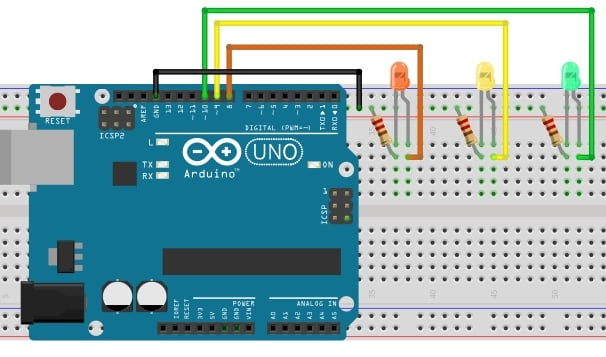### How to Run the Program

First of all, paste the code given for the Arduino in the end of this post in the Arduino IDE and upload the code.

Then you will need to download the sketch from the examples page of Wekinator

Download the source code to process a simple 10x10 color grid. Unzip it and run the code in processing. This program will use the webcam of your laptop, and depending on what you are doing in front of the camera, it will give the input to the Wekinator.

You will need another sketch for the output from the Wekinator. The code for that sketch is at the end of this post. Paste it into processing and run the sketch. This sketch will take the output from the Wekinator and send it to the Arduino, where the LEDs will light up.

Both the processing windows should look like shown below.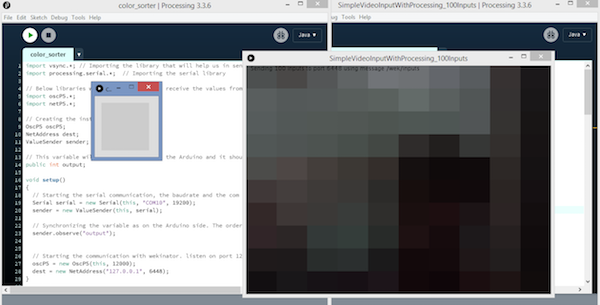Now open Wekinator and make the settings as shown in the below figure. Set the inputs to 100 and the outputs to 1. Set the type to All Classifiers with 3 classes and click on next.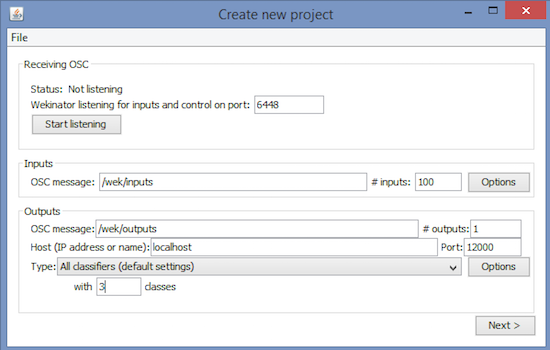Hold a colored paper in front of the webcam and start the recording for half a second.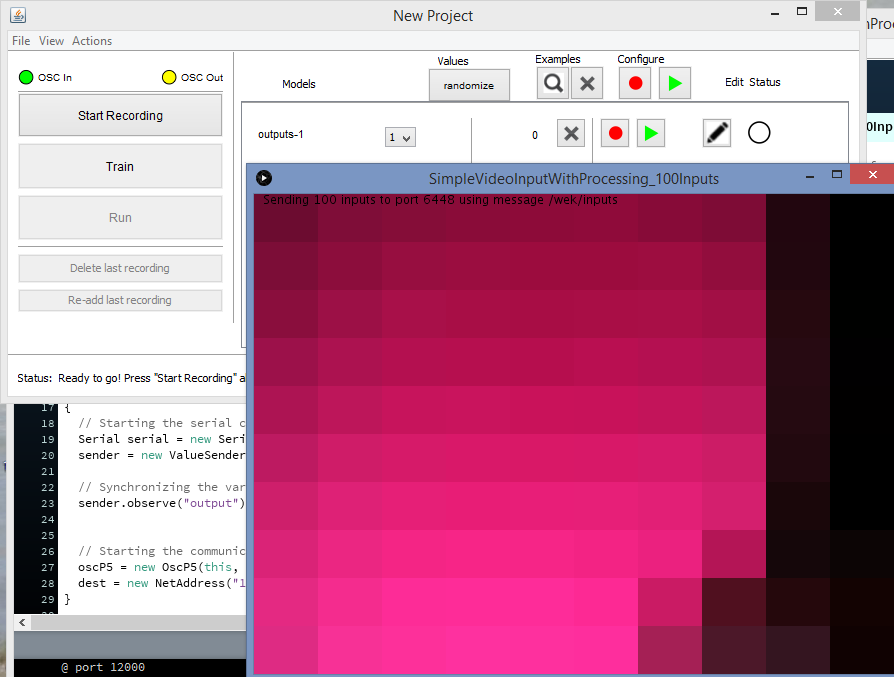Now change the class to 2. Again, show the webcam a paper of another color, and start the recording for half a second.Now change the class to 3, show a paper of yet another color, and start the recording for half a second.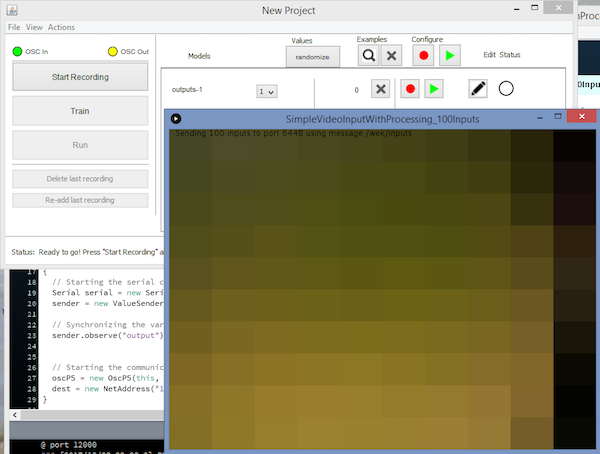After that, click “Train”, then click “Run”. Now the LEDs connected to the Arduino will light up according to the color you show in front of the webcam.

### Arduino Code

```        ```#include <VSync.h>    //Including the library that will help us in receiving and sending the values from processing
Put the number of values to synchronize in the brackets */

/* The below variable will be synchronized in the processing
and they should be same on both sides. */
int output;

// Initializing the pins for led's
int led1 = 8;
int led2 = 9;
int led3 = 10;

void setup()
{
/* Starting the serial communication because we are communicating with the
Arduino through serial. The baudrate should be same as on the processing side. */
Serial.begin(19200);

pinMode(led1, OUTPUT);
pinMode(led2, OUTPUT);
pinMode(led3, OUTPUT);

// Synchronizing the variable with the processing. The variables must be int type.
}

void loop()
{
// Receiving the output from the processing.

// Matching the received output to light up led's
if (output == 1)
{
digitalWrite(led1, HIGH);
digitalWrite(led2, LOW);
digitalWrite(led3, LOW);
}

else if (output == 2)
{
digitalWrite(led1, LOW);
digitalWrite(led2, HIGH);
digitalWrite(led3, LOW);
}

else if (output == 3)
{
digitalWrite(led1, LOW);
digitalWrite(led2, LOW);
digitalWrite(led3, HIGH);
}
}```
```

### Processing Code

```        ```import vsync.*; // Importing the library that will help us in sending and receiving the values from the Arduino
import processing.serial.*;  // Importing the serial library

// Below libraries will connect and send, receive the values from Wekinator
import oscP5.*;
import netP5.*;

// Creating the instances
OscP5 oscP5;
ValueSender sender;

// This variable will be syncronized with the Arduino and it should be same on the Arduino side.
public int output;

void setup()
{
// Starting the serial communication, the baudrate and the com port should be same as on the Arduino side.
Serial serial = new Serial(this, "COM10", 19200);
sender = new ValueSender(this, serial);

// Synchronizing the variable as on the Arduino side. The order should be same.
sender.observe("output");

// Starting the communication with Wekinator. listen on port 12000, return messages on port 6448
oscP5 = new OscP5(this, 12000);
}

// Recieve OSC messages from Wekinator
void oscEvent(OscMessage theOscMessage) {
// Receiving the output from Wekinator
float value = theOscMessage.get(0).floatValue();

// Converting the output to int type
output = int(value);
}
}

void draw()
{
//  Nothing to be drawn for this example
}```
```